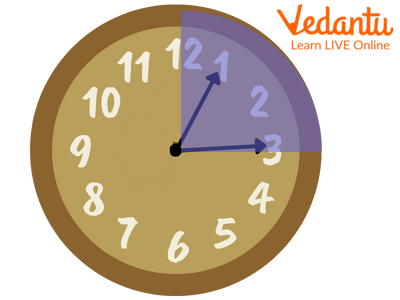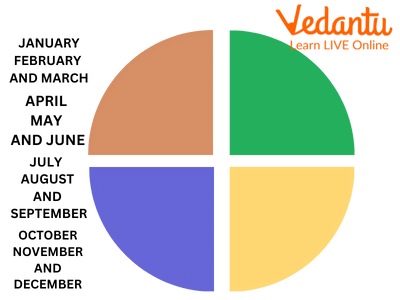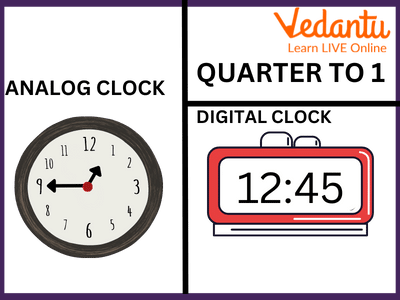Courses
Courses for Kids
Free study material
Offline Centres
More

# Learning about Quarters in a ClockLast updated date: 27th Nov 2023
Total views: 99k
Views today: 1.98k## Introduction

The time in the clock can be read in am or pm, but it can also be read as a quarter to, quarter past and half past, referring to the different time points. The clock has readings written up to 12, and the numbers are 5 minutes apart. So, we can divide the time in a way that becomes easy to read. Let us understand more about this:

### What is a Quarter?

The clock consists of 60 minutes in total which can be divided into two major parts of 30 minutes each and four minor parts consisting of 15 minutes each. The major part is called half past, and the minor part is called quarter to or quarter past. The half-past occurs when the minute hand is at 6. If the minute hand is present on 3, then it is quarter past, and if it is at 9 then it is quarter to.Quarter Past 1

• If the hour hand is at 7 and the minute hand is at 12 then we would say it is 7 o’clock.

• The minute hand will come at 3, then we would say it is quarter past 7.

• If the minute hand is present at 6 then it would be half past to 7.

• And when it would reach 9 the hour hand would be close to 8, then we would say it is quarter to 8.

### How Many Quarters in a Month?

A quarter is one-fourth of the total, whether it would be time or days in a month. To calculate the quarter of a month you can divide 30 by one-fourth. This would give you 7 and half days, which means a quarter in a month consists of 7 and half days. If a month like February is considered then it will have only 7 days in a quarter. And a month that has 31 days in total would have nearly 8 as a quarter in a month.

### How Many Quarters are in a Year?

A year has 12 months in total. To find out a quarter of a year you can divide 12 by 4 which will give you the result as 3. This means that a quarter in a year has 3 months in it.Year Divided Into Quarters

### Points To Remember

• The bigger or longer hand on the clock is the minute hand and the smaller or short hand is the hour hand.

• The minute hand from 12 takes 15 minutes to make it to quarter past.

• The minute hand from 12 takes 30 minutes to make it to half past.

• The minute hand from 12 takes 45 minutes to make it to a quarter to.Time Shown In Digital And Analog Clock

### Conclusion

An hour consists of 60 minutes which can be divided into 4 equal parts or 2 equal parts known as quarters and halves respectively. Similarly, days in a month and months in a year can also be divided into a quarter of a month and a quarter of a year.

### Sample Questions

1. How many minutes are there in a quarter of an hour?

a. 10

B. 20

c. 15

d. 30

Ans: 15

Explanation: an hour consists of 60 minutes which we can divide by 4 to get a quarter of an hour. This will result in 15. So, there are 15 minutes in a quarter of an hour.

2. What does one mean when one says it's quarter past 3?

a. 2:45

b. 3:15

c. 3:30

d. 3:45

Ans: 3:15

Explanation: Quarter past to 3 means that 15 minutes have passed since 3 o’clock. So it can be said as 3:15 too.

3. What do you mean by quarter to 11?

a. It's going to be 30 minutes from 10 o’clock

b. It's 45 minutes past 10 o’clock

c. it's going to be 12 o’clock in 15 minutes.

d. None of the above

Ans: Its 45 minutes past 10 o’clock

Explanation: Quarter to 11 means that only 15 minutes are left for 11 o’clock or in other words we can say that 45 minutes have passed since 10 o’clock and now it would be 11 o’clock soon. It can also be written as 10:45.

## FAQs on Learning about Quarters in a Clock

1. What does shorthand symbolize?

There are two hands present on the clock. The shorter hand is also known as the hour hand and it shows what hour it is going to be.

2. Can quarter past 12 be written as 11:45?

No, it cannot be written as 11:45 because a quarter past 12 means that 15 minutes have passed since 12 o’clock. This can be written as 12:15. For 11:45 it would be said as a quarter to 12 or 15 minutes are left for the clock to strike 12:00.

3. When can we say it's 4 o’clock?

The clock has two one-hour hands and the other one is the minute hand. If the minute hand is at 12 and the hour hand is at 4 then it is said to be 4 o’clock.

4. How is half past different from a quarter past?

In an hour there are 60 minutes in total if we divide it by two then we get 30 as the result which means that an hour can be divided into two equal halves of 30 minutes, each making it half past. When we divide 60 by 4 we get 15, which means an hour can be divided into 4 equal parts of 15 minutes each making it a quarter of an hour.

5. How to decide whether to use “past” or “to” in reference to time?

The minute hand decides whether to refer to as past or to. If the minute hand is moving away from 12 then it is considered as past like 10 past 3 (3:10). If the minute hand is reaching near 12 then it is referred to as “to” like 5 to 4 (3:55).